# Segment Tree Data Structure (Build and Range Query Operations)

### What is a Segment Tree?

A Segment Tree is a data structure used to answer multiple range queries on an array efficiently. Also, it allows us to modify the array by replacing an element or an entire range of elements in logarithmic time. This blog will focus on the build and query operations on segment trees.

Consider this problem: We have an array with some integers. We get multiple range queries [l, r], and the answer to each query is the minimum value from the array between the positions l and r.

Example

Suppose input array is arr[] = [3, 6, 2, 1, 5, 10, 2, 11, 4, 8], then following are the queries and their corresponding answer.

Range: [1, 2], Output: 2, Explanation: We understand from the query that we need to return the minimum value from the array between indices l = 1 and r = 2. Thus, if we look at the subarray, arr[1 : 2] = [6, 2], the minimum value in this range is 2. So the answer to this query is 2.

Range: [4, 5], Output: 1, Explanation: Similarly, for this query, we return the minimum value from the subarray arr[2 : 5] = [2, 1, 5, 10], which is 1.

### Brute force solution for Range Minimum Query problem

The brute force method to solve this problem is straightforward. We iterate from index l to r of the array for each query, finding the minimum in this range. Thus, one query takes O(r - l) time or O(n) time in the worst case. If there are q queries, this method will take O(q*n) time in the worst case. One insight is clear: as the number of queries becomes large, our code will take much time to process all queries. So our goal is to design a data structure that can answer each query in logarithmic time O(logn).

### How does Segment Tree works?

A segment tree is a binary tree such that each node stores information about a range, which depends on the problem. In our case, each node will store a minimum value in an interval. We can also use a segment tree to store different types of information such as range maximum, range sum, range XOR, etc.

Consider an array arr[] with size n and the segment tree st.

• The root of the segment tree represents the entire array i.e. arr[0 : n-1].
• Leaf nodes of st represent the single element arr[i].
• The internal nodes represent an interval arr[i : j] where 0 <= i < j < n.

We can store segment trees in an array. As we know that in a 0 based indexing of array: if a parent is present at index i, its children will be present on indices 2*i + 1, 2*i + 2. Thus the root of the tree represents the entire range of the array from 0 to n - 1.

Now we break down this interval into two half intervals. The children of the root node represent the intervals arr[0, n /2] and arr[n/2 + 1 : n - 1]. Now each interval is further divided into two halves, and the two children of each node represent these two halves. As the segment tree is divided into half in each step, the height of the segment tree will be O(logn) and the tree has n leaves corresponding to the n indices in the array.

The following image shows the segment tree for the given array. The red text represents the current node's range; the blue text is the node's indexes, and the black text is the minimum value from the array in this range.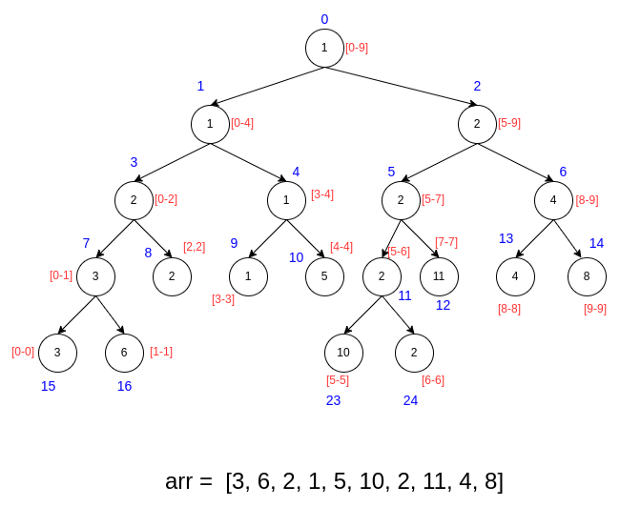## How to Build a Segment Tree for Range Minimum Queries?

### Divide and Conquer Idea of Building a Segment Tree

We use a divide and conquer strategy to build the segment tree. In the divide step, we come top-down and recursively divide the range into two halves till there is only one element in the range. We know that if there is only one element in the range, that element itself is the answer.

Now segment tree formation takes place during conquer and combine step i.e. it will start in a bottom-up fashion from the leaf nodes to the root node. In other words, it will update the nodes that come in the path from a leaf to root in this process, where data of the child nodes are used to form a parent node in each step. So, recursion will end up at the root node representing the whole array. Note: The combine step may be different for different questions like range maximum, range sum, etc.

### Divide and Conquer Steps of Building a Segment Tree

We define a function buildSegTree(int st[], int arr[], int nodeIndex, int leftRange, int rightRange) that takes these values as an input parameters:

• st[]: Segment tree array
• arr[]: Input array
• nodeIndex: Index of the current node in the segment tree.
• leftRange: Left limit of the current segment.
• rightRange: Right limit of the current segment.

Initially, nodeIndex = 0(root node), leftRange = 0, and rightRange = n - 1.

Divide Step: By calculating the mid-index, we divide the array into two halves: leftRange to mid and mid + 1 to rightRange, where mid = leftRange + (rightRange - leftRange)/2.

Conquer Step: We recursively call the same function with modified input parameters to build the segment tree for the left and right half of the array i.e. buildSegTree( 2 * nodeIndex + 1, leftRange, mid) and buildSegTree( 2 * nodeIndex + 2, mid + 1, rightRange).

Combine Step: This is the most crucial step. We use the data of the child nodes to decide what information the parent node stores. In our case, as we need to find the range minimum, we take the minimum of the values stored in the child nodes and keep them in the parent node i.e. st[nodeIndex] = min (st[2 * nodeIndex + 1], st[2 * nodeIndex + 2]). Note: If we need to find the range sum, we will take the sum of the values stored in the child nodes and store it in the parent node.

Base Case: This is the trivial case when leftRange == rightRange, i.e. this represents a single element from the array which is the situation of a leaf node. The segment tree will store this element i.e. if(leftRange == rightRange), st[nodeIndex] = arr[leftRange).

### Size of the array used in Segment Tree Construction

As discussed above, we also represent the segment tree as an array similar to the heap. The difference here is that heap is a complete binary tree structure, and segment tree is a full binary tree structure. The idea is simple: we always divide segments into two halves at every level in a segment tree.

Properties of a full binary tree:

• Every node has 0 or 2 children, and all levels are filled except the last level.
• In a full binary tree with n leaves, there will be n-1 internal nodes. So the total number of nodes will be 2*n – 1.
• Unlike a complete binary tree, the last level in a full binary tree may have gaps between nodes. So there will be some wastage of space in the simple array representation of a full binary tree. We can think to optimize this wastage, but code for segment tree operations becomes more complex.

If the size of the input array n is a power of 2, then the size of the segment tree is 2n -1. The tree has n leaf nodes and n - 1 internal nodes. Now, if we increase the input array size by 1, a new level gets added to the tree. So, if n is not a power of 2, we need to go to the next power of 2. In other words, when the array size n is not a power of 2, approximately 2k - 1 nodes are required for the segment tree, where k is the smallest power of 2 greater than n.

Example array and corresponding segment tree for finding the minimum in a range as the size of the array varies from 1 to 5. Initially, arr = , st = , size of tree = 1.arr = [1, 2], st = [1, 1, 2]

The size of tree = 3 (2n -1, where n = 2).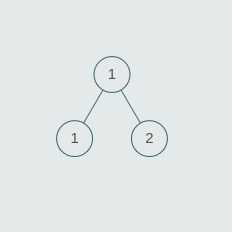Now n = 3, arr = [1, 2, 3], st =[1, 1, 3, 1, 2, null, null]

• Here n is not a power of 2.
• The smallest power of 2 greater than n = k = 4.
• So the size of the tree = 2 * k - 1 = 2 * 4 - 1 = 7.
• If we observe, the height of the tree increases by 1.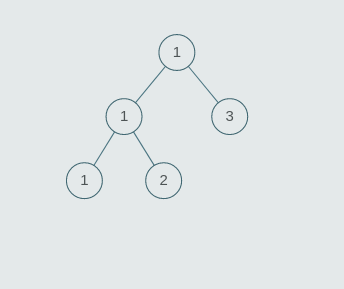arr = [1, 2, 3, 4], st = [1, 1, 3, 1, 2, 3, 4]

The size of tree = 7 (2n -1, where n = 4).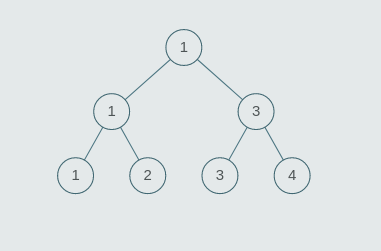arr = [1, 2, 3, 4, 5], st =[1, 1, 4, 1, 3, 4, 5, 1, 2, null, null, null, null, null, null]

• Here n is not a power of 2.
• The smallest power of 2 greater than n = k = 8.
• So the size of the tree = 2 * k - 1 = 2 * 8 - 1 = 15.
• The height of the tree again increases by 1 at this point.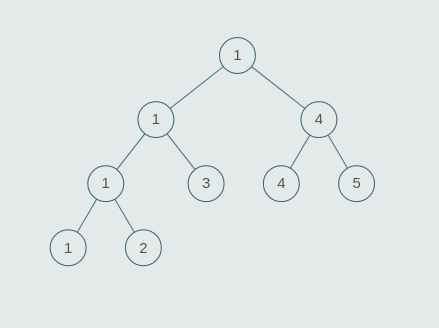### Pseudocode for Building Segment Tree

``````void buildSegTree(int st[], int arr[], int nodeIndex,
int leftRange, int rightRange)
{
if (leftRange == rightRange)
{
//leaf node
st[nodeIndex] = arr[leftRange]
}
else
{
int mid = leftRange + (rightRange - leftRange)/2

//left child
buildSegTree(st, arr, 2 * nodeIndex + 1, leftRange, mid)

//right child
buildSegTree(st, arr, 2 * nodeIndex + 2, mid + 1, rightRange)

//union of left and right, which is minimum in our case
st[nodeIndex] = min(st[2 * nodeIndex + 1], st[2 * nodeIndex + 2])
}
}``````

### Time Complexity of Building a Segment Tree

We recursively solve the problem of size n by solving the two smaller sub-problems of size n/2 and using the solutions of these two smaller problems to answer the parent node.

• The time complexity of the divide part is O(1).
• The time complexity of the conquer part is 2*T(n/2).
• The time complexity of the combine part is O(1).
• Thus, overall time complexity T(n) = 2 * T(n/2) + O(1).

We can solve this recurrence using both the recursion tree and the master method. To better understand analysis, you can refer to this blog: Analysis of recursion. If we observe closely, this recurrence relation is similar to the recurrence of the divide and conquer solution of finding max and min in an array. So the time complexity is O(n). In other words, we can build a segment tree in linear time.

### Space Complexity of Building a Segment Tree

Space complexity = Space used st[] array + Space used by recursion call stack.

• As discussed above, If the size of the input array n is a power of 2, then the size of the segment tree is 2n -1. If n is not a power of 2, then segment tree size is 2k - 1, where k is the smallest power of 2 greater than n. So overall, the size of the st[] array will be O(n).
• For the recursion call stack, space complexity is O(log n) as the stack frame size depends on the recursion tree's max depth, which is equal to O(log n).

So overall space complexity = O(n) + O(logn) = O(n)

## How does Query work in Segment Tree?

### Algorithm idea of Range Minimum Query

In this operation, we can query on a range and return the answer to the problem (minimum of the range in our case). We start from the root node of the segment tree. At each node, we check for three conditions:

• The range represented by the node is entirely inside the given range: In this case, we do not move ahead, and we return.
• The range represented by a node is completely outside the given range: In this case, we return the value stored at this node, a minimum of the range defined by the node.
• The range represented by a node is partially inside and partially outside the given range: Return the union of the values returned by the left and right nodes depending on the problem statement. We return the minimum of the left and right nodes values in our case.

### Pseudocode of Range Minimum Query

• st[]: Segment tree array
• nodeIndex: Index of the current node in the segment tree. This value will be 0 Initially.
• leftRange: Left limit of the current segment.
• rightRange: Right limit of the current segment.
• left: left limit of the given query segment.
• right: right limit of the given query segment.
``````int rangeMinQuery(int st[], int nodeIndex, int leftRange,
int rightRange, int left, int right)
{
if(right < leftRange || rightRange < left)
{
//case 2: Range represented by node is completely outside the given range
return INT_MAX
}

if(left <= leftRange && rightRange <= right)
{
//case 1: Range represented by node is completely inside the given range
return st[nodeIndex]
}

//case 3: Range represented by a node is partially inside and partially outside the given range
int mid = leftRange + (rightRange - leftRange)/2
int leftRes = rangeMinQuery(2 * nodeIndex + 1,
leftRange, mid, left, right)
int rightRes = rangeMinQuery(2 * nodeIndex + 2,
mid + 1, rightRange, left, right)
return min(leftRes, rightRes)
}``````

### Time Complexity of Range Minimum Query

To query a range minimum, we claim that we process two nodes at every level at most. We will try to prove this by contradiction. For example, consider the following tree. Suppose three nodes are explored on a level in the given segment tree.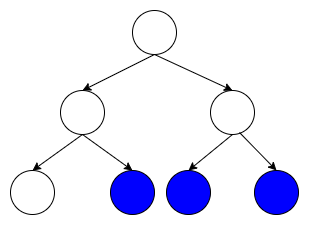The range covered by these nodes is from the leftmost colored node to the rightmost colored node. However, in this case, the range of the middle node is entirely covered. Thus, this node will immediately return the value and not be explored. Therefore, we proved that we expand at most two nodes at each level, and since there are logn levels, the number of explored nodes is 2logn = O(logn). Thus time complexity of the query operation is O(logn).

### Conclusion

In this blog, we studied how to solve multiple queries on an array in logarithmic time. We looked at the build and query operations of the segment tree. In the next blog, we will study the update operation of the segment tree.

Enjoy learning, Enjoy coding, Enjoy algorithms!

☆ 16-Week Live DSA Course
☆ 10-Week Live DSA Course